# VBA Course: Loops (exercise)

To practice what we have just learned, we'll go through the step-by-step process of creating a macro to add background colors to a 10x10 checkerboard of cells (in red and black) starting from the currently selected cell. See below: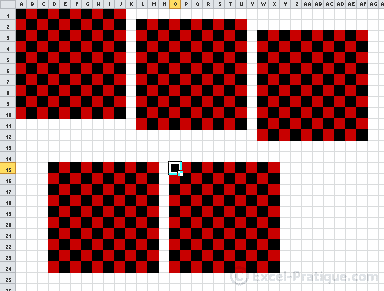Here's the first step of the exercise:

``````Sub loops_exercise()

Const NB_CELLS As Integer = 10 'Number of cells to which we want to add background colors

'...

End Sub``````

Let's start out by adding a For loop to add black backgrounds to the cells in column A (The NB_CELLS constant being 10). See below: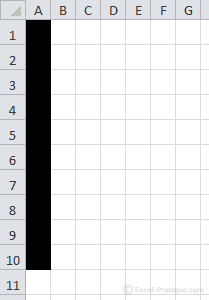Take a moment to create this loop on your own before you look at the solution ...

.
.
.
.
.
.
.
.
.
.
.
.
.
.
.
.
.
.
.
.
.
.
.
.
.
.
.
.
.
.

The solution:

``````Sub loops_exercise()

Const NB_CELLS As Integer = 10 'Number of cells to which we want to add background colors

For r = 1 To NB_CELLS 'r => row number

Cells(r, 1).Interior.Color = RGB(0, 0, 0) 'Black

Next

End Sub``````

The next step is making every other cell's background red with an If instruction (based on whether the row numbers are even or odd). See below: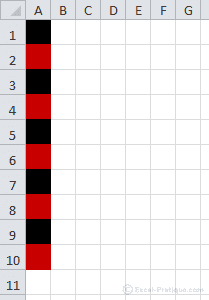.
.
.
.
.
.
.
.
.
.
.
.
.
.
.
.
.
.
.
.
.
.
.
.
.
.
.
.
.
.

The solution:

``````Sub loops_exercise()

Const NB_CELLS As Integer = 10 'Number of cells to which we want to add background colors

For r = 1 To NB_CELLS 'r => row number

If r Mod 2 = 0 Then 'Mod => is the remainder from division
Cells(r, 1).Interior.Color = RGB(200, 0, 0) 'Red
Else
Cells(r, 1).Interior.Color = RGB(0, 0, 0) 'Black
End If

Next

End Sub``````

The condition If r Mod 2 = 0 means: if the remained when we divide r by 2 equals 0 ...

Only row numbers that are even will have a remainder of 0 when they are divided by 2.

Now create a loop that executes the loop we already have for the 10 columns. See below: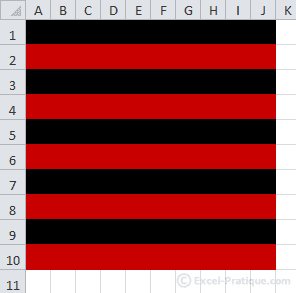.
.
.
.
.
.
.
.
.
.
.
.
.
.
.
.
.
.
.
.
.
.
.
.
.
.
.
.
.
.

The solution:

``````Sub loops_exercise()

Const NB_CELLS As Integer = 10 '10x10 checkerboard of cells

For r = 1 To NB_CELLS 'r => row number

For c = 1 To NB_CELLS 'c => column number

If r Mod 2 = 0 Then
Cells(r, c).Interior.Color = RGB(200, 0, 0) 'Red
Else
Cells(r, c).Interior.Color = RGB(0, 0, 0) 'Black
End If

Next
Next

End Sub``````

Now the second loop is nested within the first one.

To achieve this result ...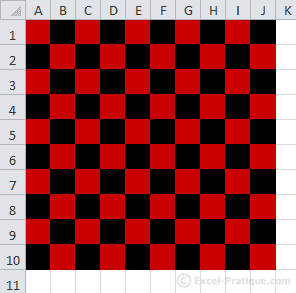Replace:

``If r Mod 2 = 0 Then``

With:

``If (r + c) Mod 2 = 0 Then``

All that's left to do is to edit the code so that the checkerboard is created starting from the currently selected cell (rather than A1). See below: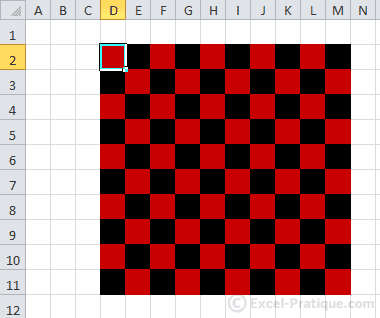.
.
.
.
.
.
.
.
.
.
.
.
.
.
.
.
.
.
.
.
.
.
.
.
.
.
.
.
.
.

The solution:

``````Sub loops_exercise()

Const NB_CELLS As Integer = 10 '10x10 checkerboard of cells
Dim offset_row As Integer, offset_col As Integer ' => adding 2 variables

'Shift (rows) starting from the first cell = the row number of the active cell - 1
offset_row = ActiveCell.Row - 1
'Shift (columns) starting from the first cell = the column number of the active cell - 1
offset_col = ActiveCell.Column - 1

For r = 1 To NB_CELLS 'Row number

For c = 1 To NB_CELLS 'Column number

If (r + c) Mod 2 = 0 Then
'Cells(row number + number of rows to shift, column number + number of columns to shift)
Cells(r + offset_row, c + offset_col).Interior.Color = RGB(200, 0, 0) 'Red
Else
Cells(r + offset_row, c + offset_col).Interior.Color = RGB(0, 0, 0) 'Black
End If

Next
Next

End Sub``````